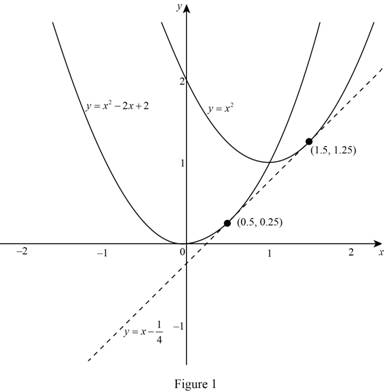# The parabolas y = x 2 and y = x 2 − 2 x + 2 and check whether there is a tangent line to both the curves or not.### Single Variable Calculus: Concepts...

4th Edition
James Stewart
Publisher: Cengage Learning
ISBN: 9781337687805### Single Variable Calculus: Concepts...

4th Edition
James Stewart
Publisher: Cengage Learning
ISBN: 9781337687805

#### Solutions

Chapter 3.1, Problem 74E
To determine

## To sketch: The parabolas y=x2 and y=x2−2x+2 and check whether there is a tangent line to both the curves or not.

Expert Solution

### Explanation of Solution

Derivative rules:

(1) Power Rule: ddx(xn)=nxn1

(2) Constant multiple rule: ddx(cf)=cddx(f)

(3) Sum rule: ddx(f+g)=ddx(f)+ddx(g)

(4) Difference rule: ddx(fg)=ddx(f)+ddx(g)

Result used:

The equation of the tangent line at (x1,y1) is, yy1=m(xx1). (1)

where, m is the slope of the tangent line at (x1,y1) and m=dydx|x=x1.

Graph:

The graph of two parabolas y=x2 and y=x22x+2 is shown below in Figure 1.From Figure 1, it is observed that there may be a line that is tangent to both the parabolas.

It is required to find the equation of the tangent line to the parabolas.

Calculation:

Consider the parabolas y=x2 and y=x22x+2.

Choose the point P(a,a2) and Q(b,b22b+2) on the parabolas y=x2 and y=x22x+2, respectively.

Suppose the slope of the required tangent line passes through the points P(a,a2) and Q(b,b22b+2), then m=b22b+2a2ba. (2)

The derivative of parabola y=x2 is dydx, which is obtained as follows.

dydx=ddx(x2)

Apply the power rule (1) and simplify the terms,

dydx=2x

Thus, the derivative of y=x2 is 2x.

Therefore, the slope of the tangent to y=x2 at (a,a2) is 2a. (3)

The derivative of parabola y=x22x+2 is dydx, which is obtained as follows.

dydx=ddx(x22x+2)

Apply the derivative rules (1), (2), (3) and (4),

dydx=ddx(x2)ddx(2x)+ddx(2)=ddx(x2)2ddx(x)+ddx(2)=2x2

Thus, the derivative of y=x22x+2 is 2x2.

Therefore, the slope of the tangent to y=x22x+2 at (b,b22b+2) is 2b2. (4)

Since the required equation of the tangent is linear from (a,a2) to (b,b22b+2), the slopes of tangent line are equal.

From equations (2) and (3), 2b2=b22b+2a2ba.

From equations (3) and (4), a=b1.

Substitute a=b1 in 2b2=b22b+2a2ba.

2b2=b22b+2(b1)2b(b1)2b2=b22b+2(b2+12b)2b2=b22b+2b21+2b2b2=1

Add 2 on both sides and obtain the value of b.

2b=1+22b=3b=32

Substitute the value b=32 in a=b1,

a=321a=12

For a=12, the slope 2a is 1 and the point P(a,a2) is (12,14).

Substitute (12,14) for (x1,y1) and 1 for m in equation (1),

y14=1(x12)y=x12+14y=x24+14y=x14

Therefore, the equation of the tangent line to the parabolas is y=x14.

### Have a homework question?

Subscribe to bartleby learn! Ask subject matter experts 30 homework questions each month. Plus, you’ll have access to millions of step-by-step textbook answers!